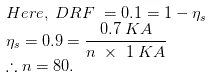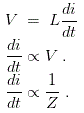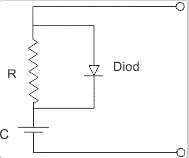# MCQs on Power Electronics

##### Page 7 of 10. Go to page 1 2 3 4 5 6 7 8 9 10
01․ A string of n parallel SCRs is operated at 72 KA, the rating of each SCR is 1 KA. If derating factor of the string is 0.1. Then calculate the value of n
60.
70.
80.
90.02․ Maximum di / dt in a SCR is
directly proportional to Vm of supply voltage.
inversely proportional to Vm of supply voltage.
inversely proportional to L in the circuit.
both A and C.03․ For series connected SCRÃ¢â‚¬â„¢s dynamic equalising circuit consists of
R and C in series but with diode across C.
R and C in series but with diode across R.
series R and diode with C across R.
series R and diode with C across R.

Dynamic equalizing circuit is Snubber circuit.04․ Anode current in an SCR consists of
holes only.
electrons only.
either electron or holes.
Both electron and holes.

As it is a bipolar device.

05․ Dynamic equalising circuit is useful
to limit di / dt of SCR.
to limit dV / dt of SCR.
for voltage equalisation.
both B and C.

Dynamic equalizing circuit is nothing but Snubber circuit.

06․ Which following is a two terminal three layer device?
BJT.
Power dioed.
MOSFET.
None of above.

The power diodes are similar to p-n junction signal or low power diodes but have large power, voltage and current handling capabilities than those of conventional p-n junction diodes. It has two terminal and three layer. It findes many application in electronics and electrical engineering circuits.

07․ Which of following is not a power transistor?
IGBTs.
COOLMOS.
TRIAC.
SITS.

The traic is a three terminal, four layer bidirectional semi conductor device. It incorporates two SCRS connected in inverse parallel with a common gate terminal in a single chip. Triac is an abbreviation for a triode ac switch. It is not a transistor (power).

08․ Which of following is normally ON device
SIT.
BJT.
TRIAC.
IGBT.

SIT stands for static induction transistor and it is a solid state version of vacuum triode valve which is used for high frequency and high power applications. SIT is normally an ON device. Electrons (majority carriers) would flow from source to n-region, pass through gate electrodes (positive) and would then continue their journey through n-, n+ and reach drain.

09․ Power transistor are type of
BJTs.
MOSFETs.
IGBTs.
All of above.

Power transistor are of four type as under (i) BJTS (ii) MOSFETs (iii) IGBTs (iv) SITs.

10․ Which of the following is true?
SIT is a high power, high frequency device.
SIT is a high power, low frequency device.
SIT is a high power, high voltage device.
SIT is a low power, high frequency device.

SIT (Static Induction Transistor) is the solid static of triode vaccum tube. It is a hogh power high frequency semiconductor device.

<<<56789>>>